# IID Deficiency

The notion of randomness, in the sense of a property of individual sequences satisfying the randomness assumption, has played a role in the foundations of probability since at least von Mises's work (see, e.g., Mises 1919). Kolmogorov's measure-theoretic axioms were more successful than von Mises's approach based on random sequences as basis for mathematical probability, but Kolmogorov still felt that von Mises's random sequences (or collectives) were indispensable for the applications of probability. In 1963 Kolmogorov made his first attempt to define the notion of randomness formally (this attempt was "incomplete", according to Kolmogorov 1965). He proposed another, much more satisfactory, definition in the last section of his 1965 paper. His new definition was based on his universal notion of complexity.

The main papers in which Kolmogorov discussed his notion of randomness were Kolmogorov (1968, 1983). The 1968 paper is an imperfect translation from Russian; the Russian version was published in 1969 in Проблемы передачи информации. In those papers Kolmogorov uses "random" in informal discussions and "Bernoulli" (adjectivally) in more formal contexts. This article uses "IID" in the same sense, since:

• "Bernoulli" is only applicable in the case of binary sequences. (Kolmogorov only considered the binary case in his papers, and discouraged his students from moving to more general cases before they understand the binary case really well.)
• "Random" is nowadays used in a very wide sense, not necessarily meaning IID (following Martin-Lof's 1966 paper).

Kolmogorov defined a binary sequence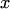of length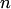and with1s to be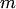-Bernoulli if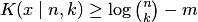, where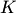is Kolmogorov complexity and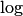is binary logarithm. Therefore,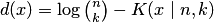measures how Bernoulli the sequenceis. However, in the modern language (see, e.g., Bienvenu et al. 2011),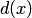is the randomness deficiency ofwith respect to the exchangeability model rather than the Bernoulli model. The randomness deficiency with respect to the class of all Bernoulli measures (see, e.g., Bienvenu et al. 2011, Section 4) is slightly different. In 1986 Kolmogorov's student (Vovk, 1986) found an exact relation between the deficiency of exchangeability (i.e, Kolmogorov's notion of the Bernoulliness deficiency) and the Bernoulliness deficiency in the modern sense. It has turned out that the latter is, essentially, equal to the former plus the binomiality deficiency of the numberof 1s in(the binomiality deficiency ofbeing defined as its randomness deficiency with respect to the class of all binomial measures on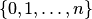). It remains an open problem to extend this result to the general observation spaces.

If the IID deficiency was computable, the problem of prediction under the randomness assumption would be straightforward. Suppose the observations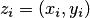consist of objects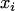and binary labels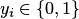. Given a training sequence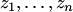and a test object, we can consider both possible completions,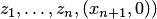and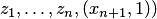. A confident prediction is possible if and only if only one of the completions has a small IID deficiency. Historically, this was the origin of conformal prediction (Vovk et al., 2005, Section 2.7).